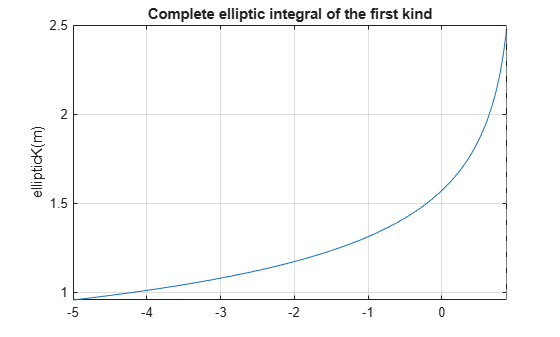Documentation

# ellipticK

Complete elliptic integral of the first kind

## Syntax

``ellipticK(m)``

## Description

example

````ellipticK(m)` returns the complete elliptic integral of the first kind.```

## Examples

### Find Complete Elliptic Integrals of First Kind

Compute the complete elliptic integrals of the first kind for these numbers. Because these numbers are not symbolic objects, you get floating-point results.

`s = [ellipticK(1/2), ellipticK(pi/4), ellipticK(1), ellipticK(-5.5)]`
```s = 1.8541 2.2253 Inf 0.9325```

Compute the complete elliptic integrals of the first kind for the same numbers converted to symbolic objects. For most symbolic (exact) numbers, `ellipticK` returns unresolved symbolic calls.

```s = [ellipticK(sym(1/2)), ellipticK(sym(pi/4)),... ellipticK(sym(1)), ellipticK(sym(-5.5))]```
```s = [ ellipticK(1/2), ellipticK(pi/4), Inf, ellipticK(-11/2)]```

Use `vpa` to approximate this result with floating-point numbers:

`vpa(s, 10)`
```ans = [ 1.854074677, 2.225253684, Inf, 0.9324665884]```

### Differentiate Complete Elliptic Integral of First Kind

Differentiate these expressions involving the complete elliptic integral of the first kind. `ellipticE` represents the complete elliptic integral of the second kind.

```syms m diff(ellipticK(m)) diff(ellipticK(m^2), m, 2)```
```ans = - ellipticK(m)/(2*m) - ellipticE(m)/(2*m*(m - 1)) ans = (2*ellipticE(m^2))/(m^2 - 1)^2 - (2*(ellipticE(m^2)/(2*m^2) -... ellipticK(m^2)/(2*m^2)))/(m^2 - 1) + ellipticK(m^2)/m^2 +... (ellipticK(m^2)/m + ellipticE(m^2)/(m*(m^2 - 1)))/m +... ellipticE(m^2)/(m^2*(m^2 - 1)) ```

### Elliptic Integral for Matrix Input

Call `ellipticK` for this symbolic matrix. When the input argument is a matrix, `ellipticK` computes the complete elliptic integral of the first kind for each element.

`ellipticK(sym([-2*pi -4; 0 1]))`
```ans = [ ellipticK(-2*pi), ellipticK(-4)] [ pi/2, Inf]```

### Plot Complete Elliptic Integral of First Kind

Plot the complete elliptic integral of the first kind.

```syms m fplot(ellipticK(m)) title('Complete elliptic integral of the first kind') ylabel('ellipticK(m)') grid on```## Input Arguments

collapse all

Input, specified as a number, vector, matrix, or array, or a symbolic number, variable, array, function, or expression.

collapse all

### Complete Elliptic Integral of the First Kind

The complete elliptic integral of the first kind is defined as follows:

`$K\left(m\right)=F\left(\frac{\pi }{2}|m\right)=\underset{0}{\overset{\pi /2}{\int }}\frac{1}{\sqrt{1-m{\mathrm{sin}}^{2}\theta }}d\theta$`

Note that some definitions use the elliptical modulus k or the modular angle α instead of the parameter m. They are related as m = k2 = sin2α.

## Tips

• `ellipticK` returns floating-point results for numeric arguments that are not symbolic objects.

• For most symbolic (exact) numbers, `ellipticK` returns unresolved symbolic calls. You can approximate such results with floating-point numbers using `vpa`.

• If `m` is a vector or a matrix, then `ellipticK(m)` returns the complete elliptic integral of the first kind, evaluated for each element of `m`.

## Alternatives

You can use `ellipke` to compute elliptic integrals of the first and second kinds in one function call.

 Milne-Thomson, L. M. “Elliptic Integrals.” Handbook of Mathematical Functions with Formulas, Graphs, and Mathematical Tables. (M. Abramowitz and I. A. Stegun, eds.). New York: Dover, 1972.

#### Mathematical Modeling with Symbolic Math Toolbox

Get examples and videos